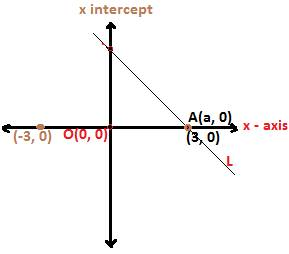## X-intercept

To understand x intercept, consider the following figure:In the above figure, there is a straight line L which passes through the point A(a, 0) on the x axis.

The x coordinate in the point A through which the straight line L passes is called the x intercept of the line L.

Unless a straight line L passes through a point lying on the x axis, the x coordinate in the point will not turn into x intercept. Therefore, x intercept exists with respect to a straight line.

Since the x intercept is x coordinate, therefore it can be both positive and negative based on the x coordinate.

If the straight line cuts the x axis at the point which lies to the right of the origin O(0, 0), then the x intercept of the line L will be positive.

For example, if a straight line L passes through the point A(3, 0), then the x intercept 3 of the Line L is positive. Look at the figure above for this.

And again, if some other straight line L’ passes through the point A (-3, 0), then the x intercept of the line L will be negative. It is because the point A (- 3, 0) lies to the left of the origin O (0, 0).

Now, we will discuss some methods for finding x intercept of straight line L based on the information given.

Method 1:

Example 1:

What is the x intercept of the straight line L having a slope 2 and passing through the point P (0, 4)?

Solution:

We know the x intercept is x coordinate in the point on the x axis trough which a straight line will pass.

So, let the point be written as A (a, 0).

Now since, the line L passes through the point P (0, 4) also besides A (a, 0), therefore, the slope formula can be applied to find the x intercept a of the line L.

From slope formula, we have

(4 – 0)/(0 – a) = 2, i.e. 4 = -2a, so a = -2

Alternative method

x intercept can also be found using the slope-intercept form of equation of a straight line L
we know, the slope-intercept form of equation of a straight line L having slope m and y intercept b is

y = mx + b

since, the line L passes through the point P (0, 4), therefore, 4 is y intercept of the line L, i,e,. b = 4 in y = mx +b

so, the equation becomes y = mx + 4.

And again, substitute the coordinates of the point A (a, 0) as the line L passes through this point.

So, we get 0 = 2 × a + 4, i.e. 2a = - 4, i.e. a = -2